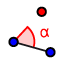# Controlling Angles with Sliders: Ex. 14

## DIRECTIONS:

1) Construct a segment . 2) Select the SLIDERtool. Select ANGLE. Set Min = . Set Max = . Set Increment = . Hit OK. Slider should be named . 3) Select the ANGLE WITH GIVEN SIZEtool. Select point B. Then select point A (vertex of soon-to-appear angle). Then, where it says , delete this and replace with (see menu off to right). Select COUNTERCLOCKWISE. If you do this correctly, you should see a new point B' so that . 4) Construct a ray with endpoint B that passes through B'. 5 )Select the ANGLE WITH GIVEN SIZE tool. Select point A. Then select point B. Then, where it says , delete this and replace with (see menu off to right). Select CLOCKWISE. You should see a new point A' so that . More directions appear below the applet.
6) Construct a ray with endpoint A that passes through A'. 7) Plot the point of intersection of the 2 rays you've just constructed in steps (4) & (6). 8) Go to the algebra view (left). Hide the 2 rays, the original segment , and the points A' and B'. 9) Use the POLYGON tool with vertices A, B, and the point of intersection of the 2 rays you constructed in step (7).

## 10)

Display the length of all sides of this triangle. Move the slider around. What do you notice? What can we conclude about a triangle with 2 congruent angles?

## 11)

What happens if we set ?

When you're done (or if you're unsure of something), feel free to check by watching the quick silent screencast below.